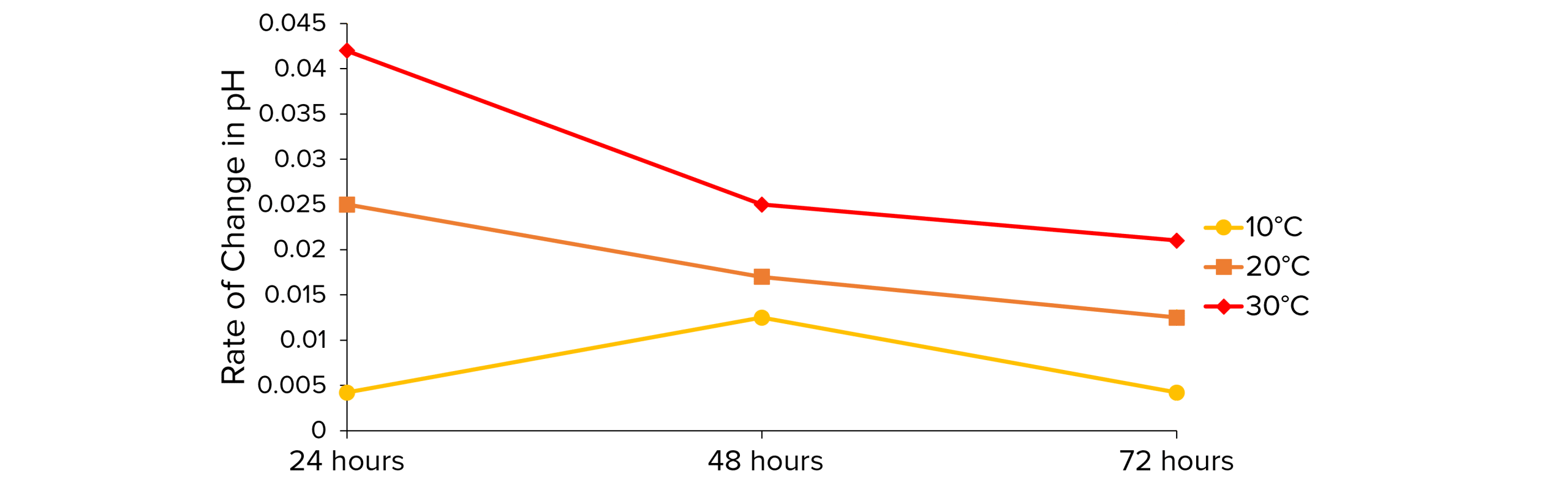# Decomposition

GCSEAQABiology FoundationBiology Higher

## Decomposition

Plant and animal waste is broken down by microorganisms called decomposers to release energy in a process called decay or decomposition. Humans take advantage of this process to make compost and biogas. You can investigate the rate of decay in a lab by measuring changes in pH.

## Compost

When organic waste is decayed by decomposers in the soil, compost is made. Compost is rich in nutrients and is used as natural fertiliser for growing plants. Farmers and gardeners will therefore create optimal conditions for the decomposers so they create compost quickly.

• The temperature affects the rate of decomposition as the process relies on enzymes. Increasing the temperature increases the rate of enzyme activity and therefore the rate of decomposition but only up to a point. If the temperature gets too hot, the enzymes start to denature, decay stops and the organism dies.
• Water is essential for lots of biological processes, including those that take place within microorganisms. The microorganisms therefore function better in moist environments.
• Certain microorganisms decompose matter aerobically (using oxygen) and some decay matter anaerobically (without oxygen). Providing the right oxygen levels for the type of decomposer can make the rate of decomposition increase.
• Increasing the number of decomposers will increases the rate at which the organic matter is decayed.
GCSEBiology FoundationBiology HigherAQA

## Biogas

Anaerobic decomposers produce methane gas as a by-product of the decay process. Methane is used in biogas which is burnt as a fuel

Biogas can be made on a large scale in biogas generators or digesters using sewage or waste from factories.

There are different types of biogas generator but they all have an inlet for the waste material, an outlet for the digested material which can be used as fertiliser and and outlet for the biogas

The biogas is collected and piped to where it is needed i.e. burnt for heating or to generate electricity. Biogas must be used straight away because it cannot be stored as a liquid.

GCSEBiology FoundationBiology HigherAQA

## Required Practical

Investigating rate of decay by measuring pH changes.

This experiment will investigate the affect of temperature on the rate of decay of milk. As milk is decayed by bacteria, the pH reduces because lactose sugar is converted into lactic acid. Therefore, measuring the pH over a period of time can help us determine the rate of decay

Doing the experiment

1. Set up 3 different water baths at different temperatures , e.g. $10\degree\text{C}$, $20\degree\text{C}$, $30\degree\text{C}$.
2. Measure out $20\text{cm}^3$ of milk into $3$ different beakers
3. Use universal indicator solution/paper or a pH probe to measure the pH of the milk in each beaker.
4. Cover each beaker in cling film and place them in separate water baths.
5. Use universal indicator solution/paper or a pH probe to check the pH of each beaker after $24\text{, } 48 \text{ and } 72 \text{ hours}$.  The pH should decrease over time.Analysing the results

The rate of decay is how much something has decayed over a period of time. Calculate the rate of decay every $24$ hours and at each temperature

1. Calculate how much the pH has changed every $24$ hours (at each temperature) by subtracting the current pH from the pH reading taken $24$ hours before2. Calculate the rate of change/decay by dividing the change in pH by the time ($24$ hours).3. You can then plot the results on a graph to show how the rate of decay changes over time and at different temperaturesGCSEBiology FoundationBiology HigherAQA

## Decomposition Example Questions

Any $2$ from:

• Temperature.
• Amount of water.
• Amount of oxygen.
• Number or decomposers.Gold Standard Education

It cannot be stored as a liquid.Gold Standard Education

$\text{Rate of decay} = \dfrac{\text{Change in pH}}{\text{Time}}$

$\text{Rate of decay} = \dfrac{6.5-4.7} {72\text{ (hours)}}$

$\text{Rate of decay} = 0.025$